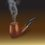An Interesting Application of Real Analysis in Geography

Does anybody here know that at any time instant there exist two antipodal points on the earth's equator that have the same temperature? The proof of this fact does not require any advanced knowledge in geography or meteorology. In fact, if we consider temperature to be a continuous function of space, then this fact can be derived from the principles of real analysis. First we shall prove the following proposition: "Let $f:[0,1]\rightarrow R$ be a continuous function such that $f(0)=f(1)$. Then $\exists c\in \left[ 0,\frac { 1 }{ 2 } \right]$ such that $f\left( c \right) =f\left( c+\frac { 1 }{ 2 } \right)$." Proving this proposition is very trivial. We simply consider the function $g\left( x \right) =f\left( x \right) -f\left( x+\frac { 1 }{ 2 } \right)$. Then $g(x)$ is continuous on $\left[ 0,\frac { 1 }{ 2 } \right]$ and we have $g\left( 0 \right) =f\left( 0 \right) -f\left( 0+\frac { 1 }{ 2 } \right) \\ =f\left( 0 \right) -f\left( \frac { 1 }{ 2 } \right) =f\left( 1 \right) -f\left( \frac { 1 }{ 2 } \right) \\ =-f\left( \frac { 1 }{ 2 } \right) +f\left( \frac { 1 }{ 2 } +\frac { 1 }{ 2 } \right) \\ =-\left\{ f\left( \frac { 1 }{ 2 } \right) -f\left( \frac { 1 }{ 2 } +\frac { 1 }{ 2 } \right) \right\} =-g\left( \frac { 1 }{ 2 } \right)$ Thus from location of roots theorem, $\exists c\in \left[ 0,\frac { 1 }{ 2 } \right]$ such that $g(c)=0$. This simply means that $f\left( c \right) =f\left( c+\frac { 1 }{ 2 } \right)$. Now we shall apply this to our given geographical proposition. Consider our equator to be the closed interval $[0,1]$ bent into a circle (this is a topological concept) with 0 and 1 as the same point on the equator. Then c and c+1/2 refer to two antipodal points. Temperature being a continuous function of the equator and temperatures at 0 and 1 being equal, we see the validity of our proposition.

For further reference to a curious reader let me tell that the generalized form of the above mathematical proposition is called Borsuk-Ulam theorem.Note by Kuldeep Guha Mazumder
3 years, 10 months ago

This discussion board is a place to discuss our Daily Challenges and the math and science related to those challenges. Explanations are more than just a solution — they should explain the steps and thinking strategies that you used to obtain the solution. Comments should further the discussion of math and science.

When posting on Brilliant:

• Use the emojis to react to an explanation, whether you're congratulating a job well done , or just really confused .
• Ask specific questions about the challenge or the steps in somebody's explanation. Well-posed questions can add a lot to the discussion, but posting "I don't understand!" doesn't help anyone.
• Try to contribute something new to the discussion, whether it is an extension, generalization or other idea related to the challenge.
• Stay on topic — we're all here to learn more about math and science, not to hear about your favorite get-rich-quick scheme or current world events.

MarkdownAppears as
*italics* or _italics_ italics
**bold** or __bold__ bold

- bulleted
- list

• bulleted
• list

1. numbered
2. list

1. numbered
2. list
Note: you must add a full line of space before and after lists for them to show up correctly
paragraph 1

paragraph 2

paragraph 1

paragraph 2

> This is a quote
This is a quote
# I indented these lines
# 4 spaces, and now they show
# up as a code block.

print "hello world"
# I indented these lines
# 4 spaces, and now they show
# up as a code block.

print "hello world"
MathAppears as
Remember to wrap math in $$...$$ or $...$ to ensure proper formatting.
2 \times 3 $2 \times 3$
2^{34} $2^{34}$
a_{i-1} $a_{i-1}$
\frac{2}{3} $\frac{2}{3}$
\sqrt{2} $\sqrt{2}$
\sum_{i=1}^3 $\sum_{i=1}^3$
\sin \theta $\sin \theta$
\boxed{123} $\boxed{123}$

Sort by:

I like it

- 3 years, 8 months ago

Thanks.

- 3 years, 8 months ago

Really interesting!

- 3 years, 10 months ago

Did you like it? In formal mathematical language, we call this Borsuk-Ulam theorem.

- 3 years, 10 months ago